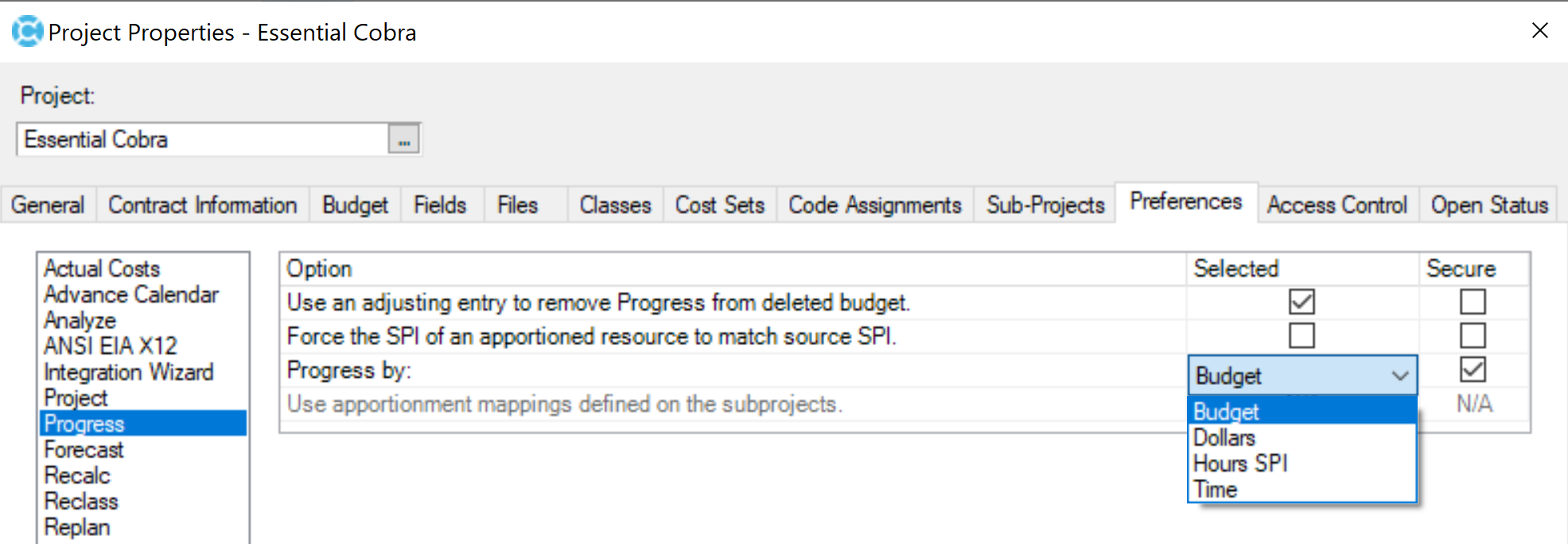# Deltek Cobra Earned Value by Budget Method

The calculation of Earned Value (EV), or performance, is central to Deltek Cobra. EV is always measured at the work package level in Cobra, and the nature of the calculation depends on the Earned Value Technique (EVT) that is used by the work package.

by Alan Kristynik, PMP

Additionally, Cobra provides a project option that lets you choose to calculate earned value by:

• Budget
• Dollars
• Time
• Hours Schedule Performance Index (SPI)

This article discusses the options available in Cobra for calculating earned value using the available Earned Value Calculation Methods that can affect how earned value calculations are performed.

#### Calculating Earned Value Methods

In addition to allowing a variety of earned value techniques (EVTs) to be assigned, Cobra provides a project preference that permits organizations to choose one of four methods:

Method Description
Budget The default method for calculating Earned Value or Progress in Cobra is by Budget Method. Cobra sums up the first result across all periods and applies the progress technique. Cobra calculates earned value by moving through the time-phased table up to the time of the calculation, earning the first result and the associated budget. If only a portion of the first result for the last period calculated is earned, Cobra uses ratios to calculate the corresponding portion of the associated budget costs.
Note: For organizations that deploy projects where the predominate and prevailing work packages are short in duration or have budgets spread relatively evenly, then the Budget Method for calculating earned value produces generally acceptable results since progress is based on hours worked rather than budgets or elapsed days.
Dollars The Dollars Method instructs Cobra to calculate Earned Value or Progress based on total budgeted currency. The currency earned is then pro-rated to arrive at the remainder of the resource costs. This method is typical of an earlier generation of Earned Value Systems developed by financial rather that scheduling organization.
Note: If you are transferring information from an external schedule to a project using the dollar method of calculating earned value, the status of the work package should be based on the budget costs earned by the activity.
Time The Time Method bases the calculation of earned value on a percentage of the work package’s time-phased budget. Thus, a work package that is 50% complete earns the portion of the work package’s budget that corresponds to the first half of that work package’s duration. This method has the advantage of forcing budgeted costs to be earned as they were originally planned, particularly in cases where work packages are assigned mixed groups of resources.
Note: If you are transferring information from an external scheduling system to a project using the time method of calculating earned value, the status of the work package should be based on the number of elapsed days for the activity.
Hours SPI The Hours SPI method is a means to back into the currency amount of earned value so that the schedule performance index (SPI) based on the hours is the same for both hours and the total currency value. To do this, Cobra calculates the earned value, or the hours based on the progress technique.
Then, Cobra calculates the SPI = EV / Budget Cumulative to Date
Finally, Cobra calculates the currency value as EV = SPI × Budget Cumulative to Date
Note: If there is no Budget Cumulative to Date, Cobra does not divide by zero.

#### Calculating Earned Value by Budget

The default methodology setting for calculating earned value in Cobra is by Budget. Selecting this option instructs Cobra to summarize the budget across all periods before applying the Earned Value Technique. Earned value is calculated by moving through the time-phase (T-Phase) table up to the time of the calculation, earning the first result and associated budget.

In most project management environments, this is typically the recommended approach for calculating Earned Value. Estimates of percent complete are typically based on the hours worked, not the amount of actual costs that have been incurred or the number of days that have elapsed since the activity was scheduled to start. Using small discrete measures of progress reduces problems with the schedule variance, which can be further reduced by limiting work packages to a single resource.

In cases where multiple resources are required, their budgets should reflect a single work effort. In other words, avoid planning work packages that include resources for dissimilar types of activities that occur at different times over the course of the work package. In other words, the budget unit is summed across all periods and the Earned Value Technique (EVT) is applied. Earned Value is calculated by moving through the T-phase Table up to the time of the calculation earning the budget unit and the associated budget. If only a portion of the budget unit for the last period calculated is earned, ratios are used to calculate the corresponding portion of the associated budget costs.

To illustrate how the Budget Method for calculating Earned Value works, assume that a work package with Planned Value (PV) with two resources have duration of two periods, assigned the 50‑50 EVT,

Resource Period 1 PV Period 2 PV Total BCWS
ENG Labor
Hours 10 10 20
Direct Dollars 50.00 75.00 125.00
MATERIAL
Direct 400.00 400.00

If the work package has started, the Earned Value for the 1st fiscal period is distributed for each resource as follows:

Resource Period 1 PV Period 2 PV Total BCWS
ENG Labor
Hours 10 20
Direct Dollars 50.00 125.00
MATERIAL
Direct 200.00 200.00

20 hours × (50 / 100) = 10 hours; \$400.00 × (50 / 100) = \$200.00

As the above example shows, one-half of the direct material dollars has been earned for the 1st period, even though the original budget spread had all the direct material dollars planned for the 2nd period.If you’re building a team and you have positions you can’t fill, you need to use SMA Talent on Demand (TOD®)! With TOD®, you can find experienced talent, such as Alan, matched to your exact needs:

Published on May 22, 2020 by